# Graphical Modeling

## Producer Field Guide

HGD_Product
Producer Field Guide
HGD_Portfolio_Suite
Producer

Use graphical modeling to draw models using tools that define inputs, functions, and outputs. This type of modeling is very similar to drawing flowcharts, in that you identify a logical flow of steps needed to perform the desired action. Through the extensive functions and operators available in the ERDAS IMAGINE graphical modeling program, you can analyze many layers of data in very few steps without creating intermediate files that occupy extra disk space. Modeling is performed using a graphical editor that eliminates the need to learn a programming language. Complex models can be developed easily and then quickly edited and re-run on another data set.

Image Processing and GIS

In ERDAS IMAGINE, the traditional GIS functions (for example, neighborhood analysis, proximity analysis, recode, overlay, index, and so forth) can be performed in models, as well as image processing functions. Both thematic and continuous layers can be input into models that accomplish many objectives at once.

For example, suppose there is a need to assess the environmental sensitivity of an area for development. An output layer can be created that ranks most to least sensitive regions based on several factors, such as slope, land cover, and floodplain. To visualize the location of these areas, the output thematic layer can be overlaid onto a high resolution, continuous raster layer (for example, SPOT panchromatic) that has had a convolution filter applied. All of this can be accomplished in a single model. Models delivered with ERDAS IMAGINE are preconfigured and editable, such as this model for vegetation analysis, shown in the figure below.

Graphical Model for Normalized Difference Vegetation Index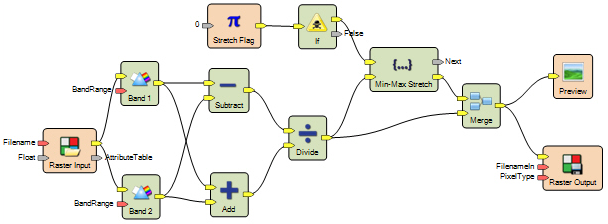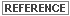Descriptions of many graphical models delivered in ERDAS IMAGINE are available in the Help.

Model Structure

A model created with Spatial Modeler or Model Maker is essentially a flow chart that defines:

• Input images, vectors, matrix or matrices, tables, and scalars to be analyzed
• Calculations, functions, or operations to be performed on the input data
• Output image, matrix or matrices, tables, and scalars to be created

The graphical models created in Spatial Modeler or Model Maker all have the same basic structure: input, function, output. The number of inputs, functions, and outputs can vary, but the overall form remains constant. All components must be connected to one another before the model can be executed. The model on the left in the figure below the most basic form. The model on the right is more complex, but it retains the same input > function > output flow.

Graphical Model Structure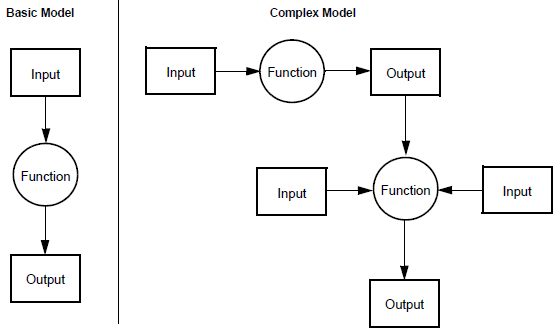Graphical models are stored in ASCII files with the .gmdx or .gmd extension. There are several sample graphical models delivered with ERDAS IMAGINE that can be used as is or edited for more customized processing.See the Help for instructions on editing existing models.

Model Maker Functions

The functions available in Model Maker are divided into the following categories:

 Category Description Analysis Includes convolution filtering, histogram matching, contrast stretch, principal components, and more. Arithmetic Perform basic arithmetic functions including addition, subtraction, multiplication, division, factorial, and modulus. Bitwise Use bitwise and, or, exclusive or, and not. Boolean Perform logical functions including and, or, and not. Color Manipulate colors to and from RGB (red, green, blue) and IHS (intensity, hue, saturation). Conditional Run logical tests using conditional statements and either...if...or...otherwise. Data Generation Create raster layers from map coordinates, column numbers, or row numbers. Create a matrix or table from a list of scalars. Descriptor Read attribute information and map a raster through an attribute column. Distance Perform distance functions, including proximity analysis. Exponential Use exponential operators, including natural and common logarithmic, power, and square root. Focal (Scan) Perform neighborhood analysis functions, including boundary, density, diversity, majority, mean, minority, rank, standard deviation, sum, and others. Focal Use Opts Constraints on which pixel values to include in calculations for the Focal (Scan) function. Focal Apply Opts Constraints on which pixel values to apply the results of calculations for the Focal (Scan) function. Global Analyze an entire layer and output one value, such as diversity, maximum, mean, minimum, standard deviation, sum, and more. Matrix Multiply, divide, and transpose matrices, as well as convert a matrix to a table and vice versa. Other Includes over 20 miscellaneous functions for data type conversion, various tests, and other utilities. Relational Includes equality, inequality, greater than, less than, greater than or equal, less than or equal, and others. Size Measure cell X and Y size, layer width and height, number of rows and columns, and so forth Stack Statistics Perform operations over a stack of layers including diversity, majority, max, mean, median, min, minority, standard deviation, and sum. Statistical Includes density, diversity, majority, mean, rank, standard deviation, and more. String Manipulate character strings. Surface Calculate aspect and degree or percent slope and produce shaded relief. Trigonometric Use common trigonometric functions, including sine and arcsine, cosine and arccosine, tangent and arctangent, hyperbolic arcsine, arccosine, cosine, sine, and tangent. Zonal Perform zonal operations including summary, diversity, majority, max, mean, min, range, and standard deviation.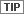These functions are also available for script modeling.See Modeler Function Categories in Spatial Modeler Language Reference Guide (Legacy) for detailed information about the available functions and operators.

Objects

Within Model Maker, an object is an input to or output from a function. The basic object types used in Model Maker are:

• raster
• vector
• matrix
• table
• scalar

Raster

A raster object is a single layer or multilayer array of pixel data. Rasters are typically used to specify and manipulate data from image files.

Vector

Vector data in either a vector coverage, shapefile, or annotation layer can be read directly into the Model Maker, converted from vector to raster, then processed similarly to raster data; Model Maker cannot write to coverages, or shapefiles or annotation layers.

Matrix

A matrix object is a set of numbers arranged in a two-dimensional array. A matrix has a fixed number of rows and columns. Matrices may be used to store convolution kernels or the neighborhood definition used in neighborhood functions. They can also be used to store covariance matrices, eigenvector matrices, or matrices of linear combination coefficients.

Table

A table object is a series of numeric values, colors, or character strings. A table has one column and a fixed number of rows. Tables are typically used to store columns from the Raster Attribute Editor or a list of values that pertains to the individual layers of a set of layers. For example, a table with four rows could be used to store the maximum value from each layer of a four layer image file. A table may consist of up to 32,767 rows. Information in the table can be attributes, calculated (for example, histograms), or defined by you.

Scalar

A scalar object is a single numeric value, color, or character string. Scalars are often used as weighting factors.

The graphics used in Model Maker to represent each of these objects are shown in the figure below.

Modeling Objects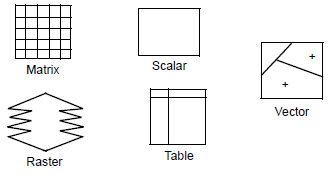Data Types

The object types described above may be any of the following data types:

• Binary—either 0 (false) or 1 (true)
• Integer—integer values from -2,147,483,648 to 2,147,483,648 (signed 32-bit integer)
• Float—floating point data (double precision)
• String—a character string (for table objects only)

Input and output data types do not have to be the same. Using SML, you can change the data type of input files before they are processed.

Output Parameters

Since it is possible to have several inputs in one model, you can optionally define the working window and the pixel cell size of the output data along with the output map projection.

Working Window

Raster layers of differing areas can be input into one model. However, the image area, or working window, must be specified in order to use it in the model calculations. Either of the following options can be selected:

• Union—the model operates on the union of all input rasters. This is the default.
• Intersection—the model uses only the area of the rasters that is common to all input rasters.

Pixel Cell Size

Input rasters may also be of differing resolution (pixel size), so you must select the output cell size as either:

• Minimum—the minimum cell size of the input layers is used (this is the default setting).
• Maximum—the maximum cell size of the input layers is used.
• Other—specify a new cell size.

Map Projection

The output map projection defaults to be the same as the first input, or projection may be selected to be the same as a chosen input. The output projection may also be selected from a projection library.

Using Attributes in Models

With the criteria function in Model Maker, attribute data can be used to determine output values. The criteria function simplifies the process of creating a conditional statement. The criteria function can be used to build a table of conditions that must be satisfied to output a particular row value for an attribute (or cell value) associated with the selected raster.

The inputs to a criteria function are rasters or vectors. The columns of the criteria table represent either attributes associated with a raster layer or the layer itself, if the cell values are of direct interest. Criteria which must be met for each output column are entered in a cell in that column (for example, >5). Multiple sets of criteria may be entered in multiple rows. The output raster contains the first row number of a set of criteria that were met for a raster cell.

Example

For example, consider the sample thematic layer, parks.img, that contains the following attribute information:

 Class Name Histogram Acres Path Condition Turf Condition Car Spaces Grant Park 2456 403.45 Fair Good 127 Piedmont Park 5167 547.88 Good Fair 94 Candler Park 763 128.90 Excellent Excellent 65 Springdale Park 548 46.33 None Excellent 0

A simple model could create one output layer that shows only the parks in need of repairs. The following logic would therefore be coded into the model:

"If Turf Condition is not Good or Excellent, and if Path Condition is not Good or Excellent, then the output class value is 1. Otherwise, the output class value is 2."

More than one input layer can also be used. For example, a model could be created, using the input layers parks.img and soils.img, that shows the soil types for parks with either fair or poor turf condition. Attributes can be used from every input file.

The following is a slightly more complex example:

If you have a land cover file and you want to create a file of pine forests larger than 10 acres, the criteria function could be used to output values only for areas that satisfy the conditions of being both pine forest and larger than 10 acres. The output file would have two classes: pine forests larger than 10 acres and background. If you want the output file to show varying sizes of pine forest, you would simply add more conditions to the criteria table.

Comparisons of attributes can also be combined with mathematical and logical functions on the class values of the input file(s). With these capabilities, highly complex models can be created.See the Help for specific instructions on using the Criteria function.